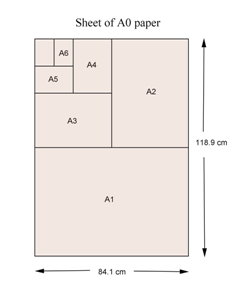# A 4 PaperAllPages RecentChanges Links to this page Edit this page Search Entry portal Advice For New UsersAll A grade sheets of paper are rectangles whose sides are in the ratio $\sqrt{2}:1.$

The area of an A0 sheet is 1 square metre.

Folding an 'Ax' sheet of paper in half produces an 'A(x+1)' sheet.

Therefore, the area of an 'An' sheet of paper is $(\frac{1}{2})^n$ square metres.

The actual dimensions of A4 are 297 mm x 210 mm. We can compute the dimensions as $\frac{250\sqrt2}{sqrt(\sqrt2)}$ mm and $\frac{250}{sqrt(\sqrt2)}$ mm that's 297.3017 ... mm and 210.2241 ... mm. Very, very close.

The ratio of sides is an irrational number, just as for a golden rectangle.